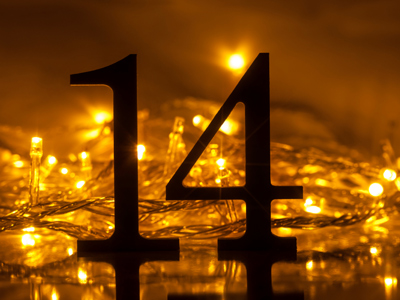Is 14 a prime number?

# Algebra - Prime Numbers

This Math quiz is called 'Algebra - Prime Numbers' and it has been written by teachers to help you if you are studying the subject at middle school. Playing educational quizzes is a fabulous way to learn if you are in the 6th, 7th or 8th grade - aged 11 to 14.

It costs only \$12.50 per month to play this quiz and over 3,500 others that help you with your school work. You can subscribe on the page at Join Us

Math students are often taught that a prime number is sometimes described as 'a number that has only two factors - 1 and itself'.

Try this Algebra quiz all about prime numbers to see if you understand what that really means.

1.
The number 24 has eight factors. Seven of the factors are 1, 2, 3, 4, 6, 12 and 24. What is the missing factor?
5
8
10
14
All the numbers given (along with the correct answer, 8) can be divided into 24 with the result that the answer is a whole number
2.
What is the next prime number after 43?
44
45
46
47
3.
Is the number 1 a prime number?
Yes, because it is a positive whole number
No
Sometimes
Yes, because it is only divisible by itself and 1
The number 1 has only one factor - itself. Remember the rule, a prime number has TWO factors itself AND 1
4.
Which of these is a prime number?
33
35
37
39
5.
What is the next prime number after 97?
98
99
100
101
1 and 101 are the only factors of 101 and therefore it is a prime number
6.
Is there an easy way to work out which numbers are prime?
Yes
No
Sometimes
Only if you are a genius
Unfortunately you have to either learn the numbers or work them out
7.
Which of these is not a prime number?
13
15
17
19
8.
Is the number 14 a prime number?
Yes
No
Yes, because it is divisible by 2
It depends which way you look at it
There are four factors of 14 - the numbers 1, 2, 7 and 14. Because there are more than two factors, it cannot be a prime number
9.
The word 'prime' is an abbreviation for what?
Primary
Primative
Priority
It is not an abbreviation
Prime is a word in its own right
10.
Which of these numbers is a prime number?
4
7
9
12
All the incorrect answers have three or more factors
Author:  Frank Evans